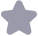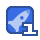### 共轭梯度法求解二元函数极小值收藏

x0=[1;1];%初始点 xk=x0; g0=zeros(2,1); g1=zeros(2,1); g2=zeros(2,1); d0=zeros(2,1); d1=zeros(2,1); syms x1 syms x2 xk1=xk; % 计算xk点的梯度及梯度值 fun=fun(x1,x2); fx1=diff(fun,'x1'); fx2=diff(fun,'x2'); fun=inline(fun); fx1=inline(fx1); fx2=inline(fx2); funval=feval&#40;fun,xk1(1&#41;,xk1(2)); gradx1=feval&#40;

...展开详情
抢沙发
一个资源只可评论一次，评论内容不能少于5个字
Ameri_关注 私信 TA的资源
上传资源赚积分,得勋章
最新推荐